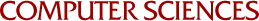## Algorithms for a Class of "Convex" Nonlinear Integer Programs

Robert Meyer, M.L. Smith
1976

Algorithms are given for the efficient solution of the class of nonlinear integer programs with separable convex objectives and totally unimodular constraints. Because of the special structure of this problem class, the integrality constraints on the variables can be easily handled. In fact, the integrality constraints actually make the problem "easier" than its continuous version, for in the case that bounds are available on the problem variables, the first of the proposed a1gorithms yields the optimal solution by the solution of a single, easily-generated linear program. For the cases in which bounds are not available for the variables or the sum of the variable ranges is very large, other algorithms are discussed that yield the solution after a finite number of linear programs and require less storage than the first algorithm.